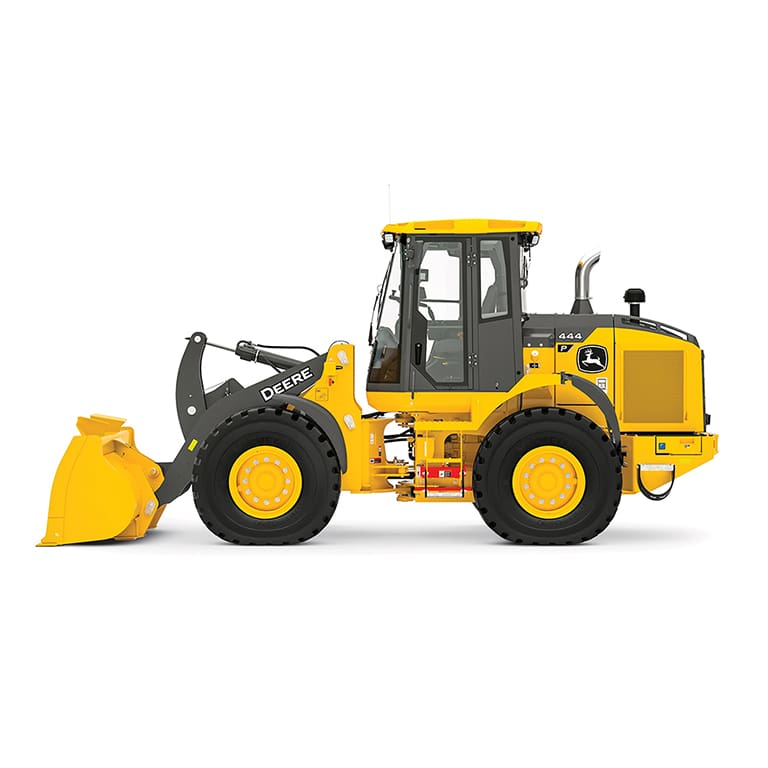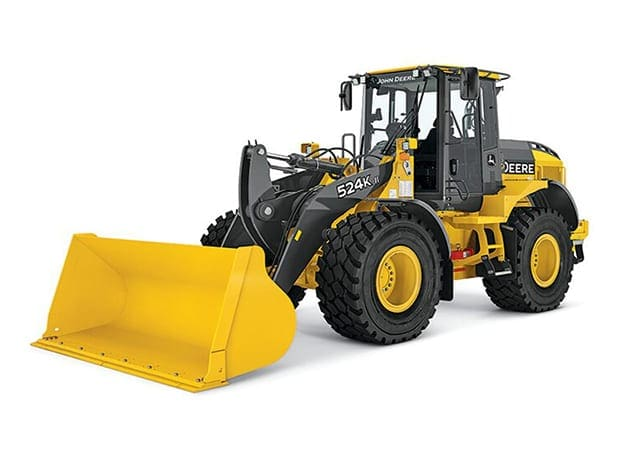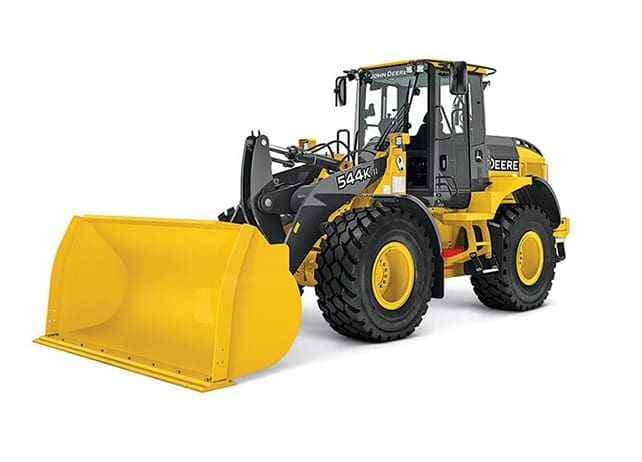## John Deere

### With enhancements including increased horsepower, ergonomic programmable controls, and reimagined electrical and hydraulic routing, combined with fuel efficient diesel engines and high-capacity heavy-duty axles, this lean, mean, hauling machine is a true 3-pass aggregate handler with payload weighing. Plus with the support of D&E, you can be certain it’ll continue to work as hard as you do.## John Deere 444 P-Tier Wheel Loader

• Engine: John Deere, PSS 4045, 4.5 L
• Emission Rating: EPA Final Tier 4/EU Stage V
• Engine Power: 92 kW (124 hp) at 2,100 rpm
• Torque: 532 Nm at 1,400 rpm
• Maximum Travel Speed:
• Forward = 35.3 km/h (Gear 4)
• Reverse = 24.6 km/h (Gear 3)
• Hydraulic Cycle Times:
• Raise = 5.8 sec
• Dump = 1.4 sec
• Lower = 4.0 sec
• Total = 11.2 sec
• Bucket Capacity: 1.9 – 3.1 m3
• Operating Weight: 11,709 kg
• Breakout Force: 8,841 kg## John Deere 524K-II Wheel Loader

• Engine: John Deere, Plus 6068H, 6.8 L
• Emission Rating: EPA Tier 3/EU Stage IIIA
• Engine Power: 106 kW (142 hp) at 1,900 rpm
• Torque: 645 Nm at 1,100 rpm
• Maximum Travel Speed:
• Forward = 38.5 km/h (Gear 5)
• Reverse = 27.6 km/h (Gear 3)
• Hydraulic Cycle Times:
• Raise = 6.1 sec
• Dump = 1.4 sec
• Lower = 3.0 sec
• Total = 10.5 sec
• Bucket Capacity: 1.9 – 2.1 – 2.3 m3
• Operating Weight: 12,622 kg
• Breakout Force: 9,638 kg

## John Deere 524 P-Tier Wheel Loader

• Engine: John Deere, PVS 6068, 6.8 L
• Emission Rating: EPA Final Tier 4/EU Stage V
• Engine Power: 113 kW (152 hp) at 1,800 rpm
• Torque: 683 Nm at 1,100 rpm
• Maximum Travel Speed:
• Forward = 40 km/h (Gear 5)
• Reverse = 27 km/h (Gear 3)
• Hydraulic Cycle Times:
• Raise = 6.1 sec
• Dump = 2.0 sec
• Lower = 4.0 sec
• Total = 12.1 sec
• Bucket Capacity: 1.9 – 2.1 – 2.3 – 3.1 m3
• Operating Weight: 13,123 kg
• Breakout Force: 11,070 kg## John Deere 544K-II Wheel Loader

• Engine: John Deere, Plus 6068H, 6.8 L
• Emission Rating: EPA Tier 3/EU Stage IIIA
• Engine Power: 122 kW (163 hp) at 1,800 rpm
• Torque: 683 Nm at 1,100 rpm
• Maximum Travel Speed:
• Forward = 40 km/h (Gear 5)
• Reverse = 29.1 km/h (Gear 3)
• Hydraulic Cycle Times:
• Raise = 5.8 sec
• Dump = 1.2 sec
• Lower = 3.2 sec
• Total = 10.2 sec
• Bucket Capacity: 2.1 – 2.3 m3
• Operating Weight: 13,121 kg
• Breakout Force: 10,115 kg

## John Deere 544 P-Tier Wheel Loader

• Engine: John Deere, PVS 6068, 6.8 L
• Emission Rating: EPA Final Tier 4/EU Stage V
• Engine Power: 123 kW (166 hp) at 1,700 rpm
• Torque: 740 Nm at 1,100 rpm
• Maximum Travel Speed:
• Forward = 40 km/h (Gear 5)
• Reverse = 29.4 km/h (Gear 3)
• Hydraulic Cycle Times:
• Raise = 5.5 sec
• Dump = 2.2 sec
• Lower = 3.5 sec
• Total = 11.2 sec
• Bucket Capacity: 1.9 – 2.1 – 2.3 – 2.5 – 3.1 m3
• Operating Weight: 13,844 kg
• Breakout Force: 13,166 kg

## John Deere 624K-II Wheel Loader

• Engine: John Deere, Plus 6068H, 6.8 L
• Emission Rating: EPA Tier 3/EU Stage IIIA
• Engine Power: 141 kW (188 hp) at 1,800 rpm
• Torque: 804 Nm at 1,400 rpm
• Maximum Travel Speed:
• Forward = 40 km/h (Gear 5)
• Reverse = 28.1 km/h (Gear 3)
• Hydraulic Cycle Times:
• Raise = 5.9 sec
• Dump = 1.3 sec
• Lower = 2.7 sec
• Total = 9.9 sec
• Bucket Capacity: 2.3 – 2.7 m3
• Operating Weight: 15,614 kg
• Breakout Force: 12,821 kg

## John Deere 624 P-Tier Wheel Loader

• Engine: John Deere, PVS 6068, 6.8 L
• Emission Rating: EPA Final Tier 4/EU Stage V
• Engine Power: 143 kW (192 hp) at 1,600 rpm
• Torque: 874 Nm at 1,300 rpm
• Maximum Travel Speed:
• Forward = 40 km/h (Gear 5)
• Reverse = 30.7 km/h (Gear 3)
• Hydraulic Cycle Times:
• Raise = 5.5 sec
• Dump = 2.0 sec
• Lower = 4.0 sec
• Total = 11.5 sec
• Bucket Capacity: 2.3 – 2.5 – 2.7 – 2.9 – 3.1 – 3.4 – 4.0 m3
• Operating Weight: 15,747 kg
• Breakout Force: 13,605 kg

## John Deere 644 G-Tier Wheel Loader

• Engine: John Deere, PVS 6068H, 6.8 L
• Emission Rating: EPA Final Tier 4/EU Stage V
• Engine Power: 169 kW (227 hp) at 1,800 rpm
• Torque: 981 Nm at 1,400 rpm
• Maximum Travel Speed:
• Forward = 37.6 km/h (Gear 4)
• Reverse = 24.6 km/h (Gear 3)
• Hydraulic Cycle Times:
• Raise = 5.2 sec
• Dump = 1.5 sec
• Lower = 3.2 sec
• Total = 9.9 sec
• Bucket Capacity: 3.1 – 3.2 m³
• Operating Weight: 18,205 kg
• Breakout Force: 14,462 kg

## John Deere 644K Wheel Loader

• Engine: John Deere, Plus 6068H, 6.8 L
• Emission Rating: EPA Tier 3/EU Stage IIIA
• Engine Power: 167 kW (224 hp) at 1,700 rpm
• Torque: 985 Nm at 1,400 rpm
• Maximum Travel Speed:
• Forward = 40 km/h (Gear 5)
• Reverse = 26.6 km/h (Gear 3)
• Hydraulic Cycle Times:
• Raise = 6.4 sec
• Dump = 1.6 sec
• Lower = 3.0 sec
• Total = 11.0 sec
• Bucket Capacity: 3.1 – 3.2 m³
• Operating Weight: 18,333 kg
• Breakout Force: 15,378 kg

## John Deere 644 P-Tier Wheel Loader

• Engine: John Deere, PSS 6068, 6.8 L
• Emission Rating: EPA Final Tier 4/EU Stage V
• Engine Power: 186 kW (249 hp) at 1,800 rpm
• Torque: 1,061 Nm at 1,400 rpm
• Maximum Travel Speed:
• Forward = 40 km/h (Gear 5)
• Reverse = 26.1 km/h (Gear 3)
• Hydraulic Cycle Times:
• Raise = 5.3 sec
• Dump = 1.4 sec
• Lower = 3.8 sec
• Total = 10.5 sec
• Bucket Capacity: 3.1 – 3.2 – 3.44 m3
• Operating Weight: 18,709 kg
• Breakout Force: 15,574 kg

## John Deere 724K Wheel Loader

• Engine: John Deere, Plus 6090H, 9.0 L
• Emission Rating: EPA Tier 3/EU Stage IIIA
• Engine Power: 197 kW (264 hp) at 1,800 rpm
• Torque: 1,159 Nm at 1,300 rpm
• Maximum Travel Speed:
• Forward = 40 km/h (Gear 5)
• Reverse = 26.6 km/h (Gear 3)
• Hydraulic Cycle Times:
• Raise = 6.4 sec
• Dump = 1.4 sec
• Lower = 3.0 sec
• Total = 10.8 sec
• Bucket Capacity: 3.2 – 3.6 m³
• Operating Weight: 19,512 kg
• Breakout Force: 15,607 kg

## John Deere 724 P-Tier Wheel Loader

• Engine: John Deere, PSS 6090, 9.0 L
• Emission Rating: EPA Final Tier 4/EU Stage V
• Engine Power: 200 kW (268 hp) at 1,800 rpm
• Torque: 1,290 Nm at 1,400 rpm
• Maximum Travel Speed:
• Forward = 40 km/h (Gear 5)
• Reverse = 26.1 km/h (Gear 3)
• Hydraulic Cycle Times:
• Raise = 5.3 sec
• Dump = 1.4 sec
• Lower = 3.8 sec
• Total = 10.5 sec
• Bucket Capacity: 3.2 – 3.4 – 3.6 – 3.8 m3
• Operating Weight: 19,825 kg
• Breakout Force: 15,988 kg

## John Deere 744 P-Tier Wheel Loader

• Engine: John Deere, PSS 6090, 9.0 L
• Emission Rating: EPA Final Tier 4/EU Stage V
• Engine Power: 236 kW (316 hp) at 1,500 rpm
• Torque: 1,501 Nm at 1,400 rpm
• Maximum Travel Speed:
• Forward = 40 km/h (Gear 5)
• Reverse = 28 km/h (Gear 3)
• Hydraulic Cycle Times:
• Raise = 5.9 sec
• Dump = 1.4 sec
• Lower = 2.8 sec
• Total = 10.1 sec
• Bucket Capacity: 3.8 – 4.0 – 4.2 – 4.5 m3
• Operating Weight: 24,894 kg
• Breakout Force: 19,414 kg

## John Deere 824 P-Tier Wheel Loader

• Engine: John Deere, PSS 6090, 9.0 L
• Emission Rating: EPA Final Tier 4/EU Stage V
• Engine Power: 256 kW (343 hp) at 1,600 rpm
• Torque: 1,627 Nm at 1,300 rpm
• Maximum Travel Speed:
• Forward = 40 km/h (Gear 5)
• Reverse = 28 km/h (Gear 3)
• Hydraulic Cycle Times:
• Raise = 5.9 sec
• Dump = 1.3 sec
• Lower = 2.5 sec
• Total = 9.7 sec
• Bucket Capacity: 3.8 – 4.0 – 4.4 – 4.6 – 4.8 – 5.2 m3
• Operating Weight: 27,056 kg
• Breakout Force: 18,720 kg

## John Deere 844 P-Tier Wheel Loader

• Engine: John Deere, PSS 6135, 13.5 L
• Emission Rating: EPA Final Tier 4/EU Stage V
• Engine Power: 311 kW (417 hp) at 1,400 rpm
• Torque: 2,119 Nm at 1,400 rpm
• Maximum Travel Speed:
• Forward = 40 km/h (Gear 5)
• Reverse = 24.7 km/h (Gear 3)
• Hydraulic Cycle Times:
• Raise = 5.9 sec
• Dump = 1.9 sec
• Lower = 3.6 sec
• Total = 11.4 sec
• Bucket Capacity: 4.8 – 5.5 – 5.7 – 6.1 m³
• Operating Weight: 33,666 kg
• Breakout Force: 22,194 kg

## John Deere 904 P-Tier Wheel Loader

• Engine: John Deere, PowerTech PSS 6135, 13.5 L
• Emission Rating: EPA Final Tier 4/EU Stage V
• Engine Power: 320 kW (429 hp) at 1,400 rpm
• Torque: 2,184 Nm at 1,400 rpm
• Maximum Travel Speed:
• Forward = 40 km/h (Gear 5)
• Reverse = 24.7 km/h (Gear 3)
• Hydraulic Cycle Times:
• Raise = 5.9 sec
• Dump = 2.3 sec
• Lower = 3.6 sec
• Total = 11.8 sec
• Bucket Capacity: 7.1 – 7.5 m3
• Operating Weight: 36,310 kg
• Breakout Force: 25,308 kg

Rugged and Reliable

360° Experience

## John Deere 644 G-Tier Wheel Loader 360° Experience

### Subscribe Now

Stay connected with our John Deere Forestry & Construction newsletter